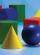# Inscribed sphere

How many percents of the cube volume takes the sphere inscribed into it?

Result

p =  52.36 %

#### Solution:Leave us a comment of example and its solution (i.e. if it is still somewhat unclear...):

Showing 0 comments:Be the first to comment!#### To solve this example are needed these knowledge from mathematics:

Our percentage calculator will help you quickly calculate various typical tasks with percentages. Tip: Our volume units converter will help you with converion of volume units.

## Next similar examples:

1. InscribedCube is inscribed in the cube. Determine its volume if the edge of the cube is 10 cm long.
2. Cone in cubeThe cube is inscribed cone. Determine the ratio of the volume of cone and cube. The ratio express as a decimal number and as percentage.
3. Sphere fallHow many percent fall volume of sphere if diameter fall 10×?
4. GasholderThe gasholder has spherical shape with a diameter 20 m. How many m3 can hold in?
5. Sphere growthHow many times grow volume of sphere if diameter rises 10×?
6. Volume of ballFind the volume of a volleyball that has a radius of 4 1/2 decimeters. Use 22/7 for π
7. PercentsHow many percents is 900 greater than the number 750?
8. Reduce of the volumeCalculate how many % reduce the volume of the cube is we reduced length of each edge by 10%.
9. Volume increaseHow many percent will increase in the pool 50 m, width 15m if the level rises from 1m to 150cm?
10. PersonsPersons surveyed:100 with result: Volleyball=15% Baseball=9% Sepak Takraw=8% Pingpong=8% Basketball=60% Find the average how many like Basketball and Volleyball. Please show your solution.
11. Highway repairThe highway repair was planned for 15 days. However, it was reduced by 30%. How many days did the repair of the highway last?
12. Frameworks is badCalculate how many percent will increase the length of an HTML document, if any ASCII character unnecessarily encoded as hexadecimal HTML entity composed of six characters (ampersand, grid #, x, two hex digits and the semicolon). Ie. space as: &#x20;
13. The ballThe ball was discounted by 10 percent and then again by 30 percent. How many percent of the original price is now?
14. Cube volumeThe cube has a surface of 384 cm2. Calculate its volume.
15. Cube surface areaThe surface of the cube was originally 216 centimeters square. The surface of the cube has shrunk from 216 to 54 centimeters sq. Calculate how much percent the edge of the cube has decreased.
16. Profit gainIf 5% more is gained by selling an article for Rs. 350 than by selling it for Rs. 340, the cost of the article is:
17. Percentages52 is what percent of 93?